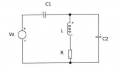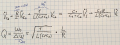# simple electric circle - understanding quality factor

#### yaron02

Joined Jan 29, 2022
2
hello every one!
There is an exercise im trying to figure out.
I've reached to following differntial equation and calculated (with parameters) the quality factor of the circle.
Now the question is "what is the physical's logic behind to result of the quality factor you just found ?"
Honestly , I dont know. (all I could tell is when R->infinity Q->0 and when R->0 Q->infinity, but i dont know what does that mean)
would any1 help ?
Here is the circle, The differential equation I found and the quality factor.#### crutschow

Joined Mar 14, 2008
29,755
Do you know what the Q of a circuit means for the characteristics of the circuit operation?

#### yaron02

Joined Jan 29, 2022
2
Do you know what the Q of a circuit means for the characteristics of the circuit operation?
well i do know it can represent how good a filter is for example.

it shows the width of the values that provide power higher than 1/2 of the maximum.

yet, idont understand why when R changes to infinity its logical that the Q is very low

#### Ian0

Joined Aug 7, 2020
5,064
well i do know it can represent how good a filter is for example.
Is this homework?

There are various definitions of Q, all of which are true, but if you get the one most relevant to the context it does make things easier to understand.

Firstly get out of you head any idea that High Q = good, low Q = bad. Better just to call it Q and forget about the term "Quality factor"

Q is the ratio of the energy stored to the energy lost per cycle.

A pendulum is a useful example. A pendulum with a high Q will keep on oscillating for a long time. One with a low Q will slow down and stop.
The same applies to your filter.
Every time current flows through the resistor some of it is turned into heat. Energy is lost and eventually the oscillation will stop.

More often than not, storing a lot of energy and oscillating at a certain frequency is not what you want a filter to do - that's the booming bass you get at a certain frequency from cheap loudspeakers, and a low Q is much better.

#### crutschow

Joined Mar 14, 2008
29,755
when R changes to infinity its logical that the Q is very low
In your circuit, with infinite R, the inductor is not carrying any current, so it's as if you have no inductor.

•DickCappels